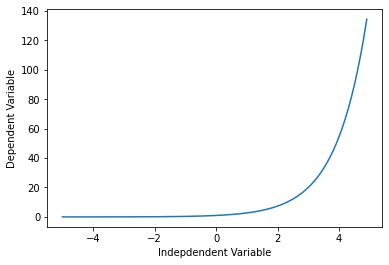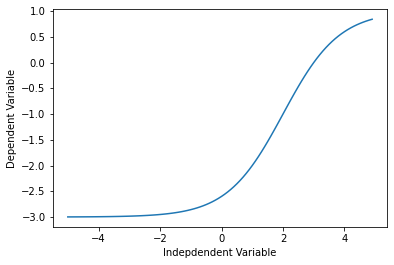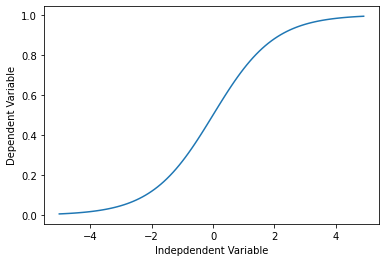# Non Linear Regression Analysis

If the data shows a curvy trend, then linear regression will not produce very accurate results when compared to a non-linear regression because, as the name implies, linear regression presumes that the data is linear. Let's learn about non linear regressions and apply an example on python. In this notebook, we fit a non-linear model to the datapoints corrensponding to China's GDP from 1960 to 2014.

## Importing required libraries

import numpy as np
import matplotlib.pyplot as plt
%matplotlib inline


Though Linear regression is very good to solve many problems, it cannot be used for all datasets. First recall how linear regression, could model a dataset. It models a linear relation between a dependent variable y and independent variable x. It had a simple equation, of degree 1, for example y = $2x$ + 3.

x = np.arange(-5.0, 5.0, 0.1)

##You can adjust the slope and intercept to verify the changes in the graph
y = 2*(x) + 3
y_noise = 2 * np.random.normal(size=x.size)
ydata = y + y_noise
#plt.figure(figsize=(8,6))
plt.plot(x, ydata,  'bo')
plt.plot(x,y, 'r')
plt.ylabel('Dependent Variable')
plt.xlabel('Indepdendent Variable')
plt.show()Non-linear regressions are a relationship between independent variables $x$ and a dependent variable $y$ which result in a non-linear function modeled data. Essentially any relationship that is not linear can be termed as non-linear, and is usually represented by the polynomial of $k$ degrees (maximum power of $x$).

$$\ y = a x^3 + b x^2 + c x + d \$$

Non-linear functions can have elements like exponentials, logarithms, fractions, and others. For example: $$y = \log(x)$$

Or even, more complicated such as : $$y = \log(a x^3 + b x^2 + c x + d)$$

Let's take a look at a cubic function's graph.

x = np.arange(-5.0, 5.0, 0.1)

##You can adjust the slope and intercept to verify the changes in the graph
y = 1*(x**3) + 1*(x**2) + 1*x + 3
y_noise = 20 * np.random.normal(size=x.size)
ydata = y + y_noise
plt.plot(x, ydata,  'bo')
plt.plot(x,y, 'r')
plt.ylabel('Dependent Variable')
plt.xlabel('Indepdendent Variable')
plt.show()As you can see, this function has $x^3$ and $x^2$ as independent variables. Also, the graphic of this function is not a straight line over the 2D plane. So this is a non-linear function.

Some other types of non-linear functions are:

$$Y = X^2$$

x = np.arange(-5.0, 5.0, 0.1)

##You can adjust the slope and intercept to verify the changes in the graph

y = np.power(x,2)
y_noise = 2 * np.random.normal(size=x.size)
ydata = y + y_noise
plt.plot(x, ydata,  'bo')
plt.plot(x,y, 'r')
plt.ylabel('Dependent Variable')
plt.xlabel('Indepdendent Variable')
plt.show()### Exponential

An exponential function with base c is defined by $$Y = a + b c^X$$ where b ≠0, c > 0 , c ≠1, and x is any real number. The base, c, is constant and the exponent, x, is a variable.

X = np.arange(-5.0, 5.0, 0.1)

##You can adjust the slope and intercept to verify the changes in the graph

Y= np.exp(X)

plt.plot(X,Y)
plt.ylabel('Dependent Variable')
plt.xlabel('Indepdendent Variable')
plt.show()### Logarithmic

The response $y$ is a results of applying logarithmic map from input $x$'s to output variable $y$. It is one of the simplest form of log(): i.e. $$y = \log(x)$$

Please consider that instead of $x$, we can use $X$, which can be polynomial representation of the $x$'s. In general form it would be written as
\begin{equation} y = \log(X) \end{equation}

X = np.arange(-5.0, 5.0, 0.1)

Y = np.log(X)

plt.plot(X,Y)
plt.ylabel('Dependent Variable')
plt.xlabel('Indepdendent Variable')
plt.show()

/usr/local/lib/python3.6/dist-packages/ipykernel_launcher.py:3: RuntimeWarning: invalid value encountered in log
This is separate from the ipykernel package so we can avoid doing imports until### Sigmoidal/Logistic

$$Y = a + \frac{b}{1+ c^{(X-d)}}$$

X = np.arange(-5.0, 5.0, 0.1)

Y = 1-4/(1+np.power(3, X-2))

plt.plot(X,Y)
plt.ylabel('Dependent Variable')
plt.xlabel('Indepdendent Variable')
plt.show()# Non-Linear Regression example

For an example, we're going to try and fit a non-linear model to the datapoints corresponding to China's GDP from 1960 to 2014. We download a dataset with two columns, the first, a year between 1960 and 2014, the second, China's corresponding annual gross domestic income in US dollars for that year.

import numpy as np
import pandas as pd

!wget -nv -O china_gdp.csv https://s3-api.us-geo.objectstorage.softlayer.net/cf-courses-data/CognitiveClass/ML0101ENv3/labs/china_gdp.csv


2020-04-27 22:31:54 URL:https://s3-api.us-geo.objectstorage.softlayer.net/cf-courses-data/CognitiveClass/ML0101ENv3/labs/china_gdp.csv [1218/1218] -> "china_gdp.csv" 

Year Value
0 1960 5.918412e+10
1 1961 4.955705e+10
2 1962 4.668518e+10
3 1963 5.009730e+10
4 1964 5.906225e+10
5 1965 6.970915e+10
6 1966 7.587943e+10
7 1967 7.205703e+10
8 1968 6.999350e+10
9 1969 7.871882e+10

### Plotting the Dataset

This is what the datapoints look like. It kind of looks like an either logistic or exponential function. The growth starts off slow, then from 2005 on forward, the growth is very significant. And finally, it decelerate slightly in the 2010s.

plt.figure(figsize=(8,5))
x_data, y_data = (df["Year"].values, df["Value"].values)
plt.plot(x_data, y_data, 'ro')
plt.ylabel('GDP')
plt.xlabel('Year')
plt.show()### Choosing a model

From an initial look at the plot, we determine that the logistic function could be a good approximation, since it has the property of starting with a slow growth, increasing growth in the middle, and then decreasing again at the end; as illustrated below:

X = np.arange(-5.0, 5.0, 0.1)
Y = 1.0 / (1.0 + np.exp(-X))

plt.plot(X,Y)
plt.ylabel('Dependent Variable')
plt.xlabel('Indepdendent Variable')
plt.show()The formula for the logistic function is the following:

$$\hat{Y} = \frac1{1+e^{\beta_1(X-\beta_2)}}$$

$\beta_1$: Controls the curve's steepness,

$\beta_2$: Slides the curve on the x-axis.

### Building The Model

Now, let's build our regression model and initialize its parameters.

def sigmoid(x, Beta_1, Beta_2):
y = 1 / (1 + np.exp(-Beta_1*(x-Beta_2)))
return y


Lets look at a sample sigmoid line that might fit with the data:

beta_1 = 0.10
beta_2 = 1990.0

#logistic function
Y_pred = sigmoid(x_data, beta_1 , beta_2)

#plot initial prediction against datapoints
plt.plot(x_data, Y_pred*15000000000000.)
plt.plot(x_data, y_data, 'ro')

[<matplotlib.lines.Line2D at 0x7fb509b1fba8>]Our task here is to find the best parameters for our model. Lets first normalize our x and y:

# Lets normalize our data
xdata =x_data/max(x_data)
ydata =y_data/max(y_data)


#### How we find the best parameters for our fit line?

we can use curve_fit which uses non-linear least squares to fit our sigmoid function, to data. Optimal values for the parameters so that the sum of the squared residuals of sigmoid(xdata, *popt) - ydata is minimized.

popt are our optimized parameters.

from scipy.optimize import curve_fit
popt, pcov = curve_fit(sigmoid, xdata, ydata)
#print the final parameters
print(" beta_1 = %f, beta_2 = %f" % (popt, popt))

 beta_1 = 690.453017, beta_2 = 0.997207


Now we plot our resulting regression model.

x = np.linspace(1960, 2015, 55)
x = x/max(x)
plt.figure(figsize=(8,5))
y = sigmoid(x, *popt)
plt.plot(xdata, ydata, 'ro', label='data')
plt.plot(x,y, linewidth=3.0, label='fit')
plt.legend(loc='best')
plt.ylabel('GDP')
plt.xlabel('Year')
plt.show()## Practice

Can you calculate what is the accuracy of our model?

# split data into train/test
msk = np.random.rand(len(df)) < 0.8
train_x = xdata[msk]
test_x = xdata[~msk]
train_y = ydata[msk]
test_y = ydata[~msk]

# build the model using train set
popt, pcov = curve_fit(sigmoid, train_x, train_y)

# predict using test set
y_hat = sigmoid(test_x, *popt)

# evaluation
print("Mean absolute error: %.2f" % np.mean(np.absolute(y_hat - test_y)))
print("Residual sum of squares (MSE): %.2f" % np.mean((y_hat - test_y) ** 2))
from sklearn.metrics import r2_score
print("R2-score: %.2f" % r2_score(y_hat , test_y) )

Mean absolute error: 0.02
Residual sum of squares (MSE): 0.00
R2-score: 0.91